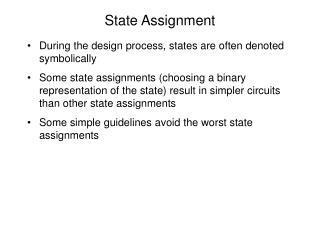# State Assignment - PowerPoint PPT PresentationDownload PresentationState Assignment

State Assignment
Download Presentation## State Assignment

- - - - - - - - - - - - - - - - - - - - - - - - - - - E N D - - - - - - - - - - - - - - - - - - - - - - - - - - -
##### Presentation Transcript

1. State Assignment • During the design process, states are often denoted symbolically • Some state assignments (choosing a binary representation of the state) result in simpler circuits than other state assignments • Some simple guidelines avoid the worst state assignments

2. Rules for State Assignment • Section 15.8 of Roth • 1) States which have the same next state for a given input should be adjacent • (adjacent means differing by a single bit in the state assignment= adjacent in a Karnaugh map) • 2) States which are the next state of a given state should be adjacent • To minimize the output equation: States which have the same output for a given input should be adjacent

3. Applying the Rules for State Assignment • Apply rule 1 first, then apply rule 2 • Multiple requirements should be placed as groups of 4 or more on the Karnaugh map • State adjacencies which occur more than once should be give precedence

4. State Transition Table for a vending machine selling a 25 cent item 5 states need 3 f-f’s How many flip-flops are required? States which have the same next state should be adjacent Examine the columns Rule 1: (D,E) (A,B,C,D,E) States which are the next state of a given state should be adjacent Examine the rows Rule 2: (A,B,C) (A,B,C,D) (A,C,D,E) (A,D,E) (A,E)

5. A B E D C Rule 1: (D,E) (A,B,C,D,E) Rule 2: (A,B,C) (A,B,C,D) (A,C,D,E) (A,D,E) (A,E) Rule 1: all 5 adjacent -- put them in a group of 4 with 1 adjacent D must be adjacent to E Rule 2: AC occurs 3 times, AD occurs 3 times, AE occurs 3 times AB occurs 2 times, BC occurs 2 times, CD occurs 2 times CE occurs 1 time q2q1 q0 Note: if D and E are adjacent, A cannot be adjacent to both D and E

6. q2q1 q0 A A B B E D C q2q1 q0 E D C Good/Bad Good Bad Note that to confirm that “Good” is better than “Bad” would require finding 6 (or 12) 5-variable minimum expressions

7. State Transition Table for a vending machine selling a 25 cent item Minimizing the output equation: States that have the same output for a given input should be adjacent. For the vend/don’t vend: (ABCDE), (ABCD), (ABC), (ABCDE) Conclusion: ABCD should be in a group of 4